Geeks With Blogs
Buhay Programmer Dont byte more than you can chew

The Goal

Simulate a class using the Record type

How we'll do this

Create a tool that can translate a number into another format. In particular, we'll convert a number into

1. Roman Numerals or
2. multiply it by 10

Why are you doing this?

No particular reason. I was just curious.  I was just thinking that if F# did not support OOP, how can I still achieve the same things I was used to doing in C#?

Ok so here we go..

F# record types are simple named types. We can pattern match over it and it is also constructed quite easily. For example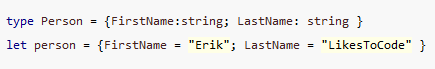The record Person contains 2 named fields, which are both strings. Most of the time records are used to hold data but since F# is an FP language, we can also have the fields of the record carry a function.  For example, we can modify the Person record type to instead of having the "LastName" as a string, we'll turn it into a function that computes the last name. Something like,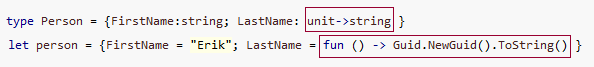As you can see we have changed the signature of LastName from "string" to "unit->string". The function "fun -> Guid.NewGuid().ToString() " matches this new signature. (Side note: as a C# coder, this was a big mind shift for me)

Going back to our example, lets define a Converter record type asthis type holds a string field "x" and "ToNewFormat" field with the signature unit->string.  The function does not take any parameter because we'll have it use the value assigned to "x" i.e. it will convert the value in "x" into a different format.  If we were using a class, our code (C#) will be something like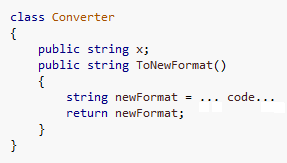and to use it we'd writeSo how do we contsruct our F# Converter record so that it would behave essentially like the C# Converter class?

like this.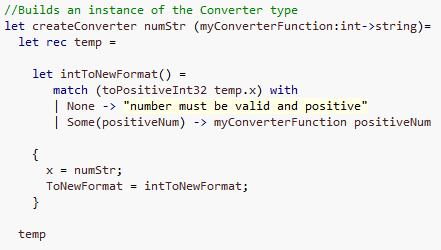We create a converter function that takes 2 parameters, "numStr" and "myConverterFunction".  NumStr gets assigned to the field "x" while the myconverterFunction is wrapped inside the "intToNewFormat" function which has the signature unit->string.

MyConverterFunction was wrapped inside intToNewFormat function because we want this function to work on the value of field "x".  Well actually myConverter function uses the value of "positiveNum" (an int) which was derived from the string value of temp.x.  The "temp" value is of course of type Converter.

The important part here is that in order for us to acces the instance value of "x" we need add the "rec" keyword in the definition of the value temp.  If we didn't do that we won't be able to access temp.x!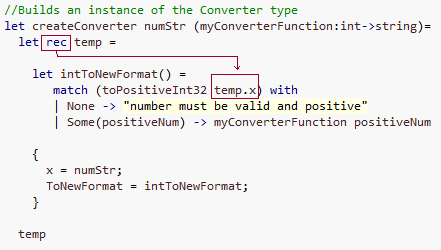To call this createConverter function, all we need to do is pass a string and a function that has the signature int->string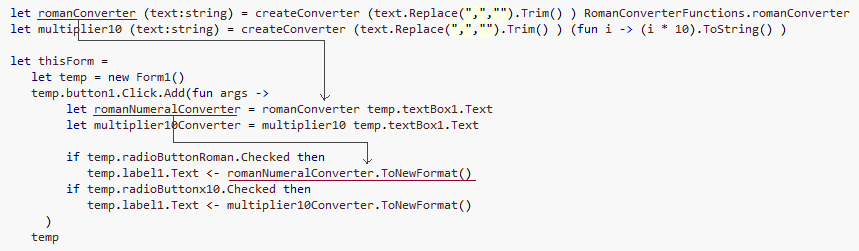In the code above, we create a romanConverter function that takes a string and creates the instance of the Converter record by passing the string and "RomanConverterFunction.romanConverter" function. The "RomanConverterFunction.romanConverter" function takes an integer and converts it to Roman numerals. For example, if you pass it "1980" it will output "MCMLXXX"

That's it! whenever we call, romanNumeralConverter.ToNewFormat(), it is converting its instance value of "x" into roman numerals exactly the same way the C# Converter class is working.

Note that because we are passing around functions we can easily create different kinds converters.  In the above code we created another converter, "multiplier10converter" which merely multiplies a number by 10 (yes, this example is not very good. :-p ).  If we needed a different converter all we need to do is write the code for that converter and pass the new conversion function to "createConverter". Simple.  If we had to do this in OO, we have to through the inheritance-override route.

Here's the tool in action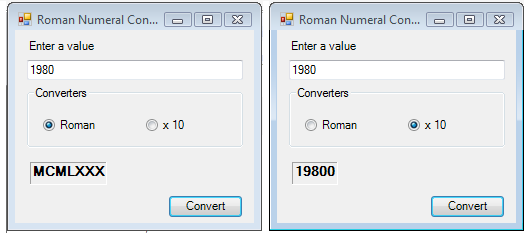Comments on this post: F#: Making records behave like objects

# re: F#: Making records behave like objects*OR* you could use the "with" keyword, which allows adding members to a record (or even a discriminated union).

Like

type Person = {FirstName: string; LastName: string} with member this.FullName() = this.FirstName + " " + this.LastName;
Left by rsenna on Feb 28, 2009 2:05 AM